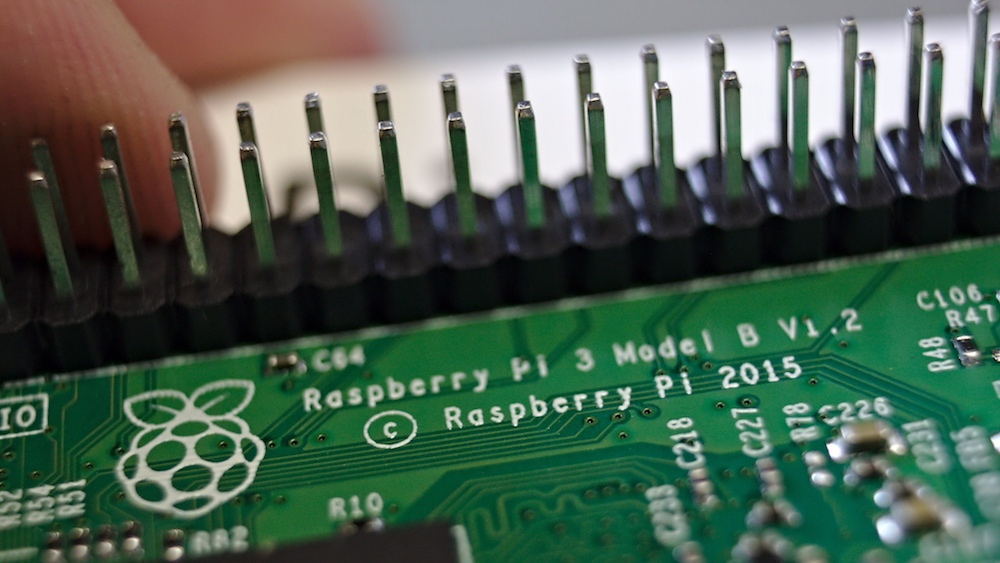Raspberry Pi

# How to Get Started With the Raspberry Pi GPIO Python LibraryAugust 29, 2018 by Robin Mitchell
ShareLearn how you can use the Raspberry Pi GPIO  Python library to handle the I/O pins on your Raspberry Pi.

## Hardware

 1 Raspberry Pi

## Software

 1 Raspbian 1 RPi.GPIO Library

GPIO on the Raspberry Pi is what makes the machine special; it allows a moderately powerful microprocessor to directly talk to circuits and interact with them. Handling I/O pins is often a very difficult task but, thankfully, there are Python libraries available that make it a trivial task.

Before you continue with this tutorial, read  How to Use Python With Raspberry Pi to get correctly set up!

### How to Import the RPi.GPIO Library

Before you can access the GPIO you need to import the correct library. At the top of your empty Python program enter the following line:

``import RPi.GPIO as GPIO``

This line imports the RPi.GPIO library and gives it the name GPIO (making it easier to access in your program).

### How to Configure the GPIO Mode

Now that the library has been imported, you need to configure it to use a numbering scheme for the pins.

If you look at the pinout of the Raspberry Pi GPIO pins you will see that each pin has a number and a name (for example, pin 12 is called GPIO18 and pin 7 is called GPIO4). This means you have two numbering schemes available and will need to pick one of them.

The first numbering scheme, BOARD, refers to pin numbers (such as pin 3, 5, 7 etc) and the second numbering scheme, BCM, refers to the pin names (GPIO4, GPIO18 etc). Which scheme you go for completely depends on your preference but BCM tends to be the more commonly used numbering system. So, for this example, set the GPIO library to use the BCM numbering scheme using the code below:

``GPIO.setmode(GPIO.BCM)``

With this scheme, the number refers to the GPIO pin. So if you use GPIO number 4, you are referring to pin number 7 on the I/O header.

### Input Pins

To read the digital state of external circuits, you can configure GPIO pins to be inputs. To do this, use the following code (note that the number 4 refers to the GPIO number â€” you can change this):

``````# This will configure GPIO04 (pin 7) to be an input
GPIO.setup(4, GPIO.IN)``````

Reading from input pins is really easy when using the function GPIO.input(pinNumber) and this function returns either a 1 or 0 depending on the logical state of the input (1 = 3.3V and 0 = 0V).

``inputValue = GPIO.input(4)		# Read logical state on GPIO04``

### Output Pins

Output pins allow you to set the voltage to either 3.3V (1) or 0V (0) which can be used to control external circuits (be warned, output pins can only source a small amount of current, so you may need to use driver circuits). Configuring a GPIO pin to be an output is easy. The following code configures GPIO 4 to be an output:

``GPIO.setup(4, GPIO.OUT)		# Configure GPIO04 as output``

Now that GPIO04 is configured as an output you can write values to it. If you want the output to be 3.3V (1) then write â€œ1â€ to it and if you want the output to be 0V (0) then write â€œ0â€ to it. The example below shows how to do this.

``````GPIO.output(4, 1)			# Output 3.3V on GPIO04 (pin 7)
GPIO.output(4, 0)			# Output 0 on GPIO04 (pin 7)``````

### AuthorRobin Mitchell

Graduated from the University Of Warwick in Electronics with a BEng 2:1 and currently runs MitchElectronics.